New Students Offer - Use Code HELLO

# Basics of Geometry PDF

Geometry is a very important topic the aspirants who are preparing for the SSC, IBPS, SBI exams. One can expect good number of questions from this topic. Now let us discuss the basic concepts which an aspirant should be clear before solving the concepts. Please make sure that all the basic concepts are clear before solving the problems otherwise it will be difficult to solve an easy problem.

THE MORE YOU IN GEOMETRY THE MORE YOU SCORE.

## POINT:

A point is the basic unit of the geometry which is visually represented by a DOT ( . ) . Also denoted by Capital letters for example.
Ex: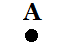#### COLLINEAR POINTS:

Two Or more points lie on the same line and in the same plane are known as collinear points.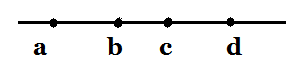Points A,b,c,d are lying on the straight line in the same plane and thus known as collinear points.

#### NON-COLLINEAR POINTS:

Points which do not lie on the same line are known are Non-collinear points.Points x,y,z,a are not lying on the same line but are on the same plane, thus they are known as non-collinear points.

#### PLANE:

A plane is a two-dimensional figure that extends infinitely.

## LINE:

A line is formed by joining number of points which are collinear.Line with arrow marks on both sides can be extended infinitely. A line is defined by its length but has no breadth.This is how we can represent a line.

#### LINE SEGMENT:

A line segment is also made up of collinear points but it has a definite starting and ending point. It is visually represented as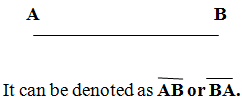It can be denoted as AB or BA.

#### RAYS:

A ray is a line with an end point and extending in one direction infinitely. Let us see how a ray looks like.ray is always represented in this way- First letter is the ending point (A).

#### CONCURRENT LINES:

Three or more lines pass through same point are called concurrent lines and the common point is known as point of concurrence.In the above figure a,b,c,d,e are the lines intersecting at Point ‘O’. Point ‘O’ is known as point of concurrence and all these lines are known as concurrent lines.

#### PARALLEL LINES:

Two lines in the same plane are said to be parallel if they never meet even though they are extended in either direction. They remain same distant for whole length. It is denoted by॥

When a line cuts the two parallel lines then it is known as transversal.AB and YZ are the two parallel lines and PQ is the transversal which intersects both AB and YZ.

Transversal makes 4 pairs of -CORRESPONDING ANGLES, Which are equal in value. They areTransversal makes 4 pairs of ALTERNATE ANGLES which are equal*Angles 3,4,5,6 are known as the interior angles. Sum of the interior angles on the same side of the transversal is equal to two right angles i.e 180˚.*Angles 1,2,7,8 are known as the exterior angles. Sum of the exterior angles on the same side also equal to two right angles i.e 180˚.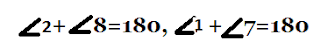#### PERPENDICULAR LINES:

When two rays are joined with a common end point, then an angle is formed. The common end point is called as the vertex of the angle. The rays are known as the sides of the angle.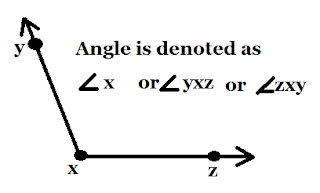#### Types of Angles: Angles based on the value of the angle.

Acute Angle: An angle whose value lies between 0˚ and 90˚ is called as an acute angle.
Right Angle: An angle whose value is 90˚ is called right angle.
Obtuse Angle: An angle whose value lies between 90˚ and 180˚ is known as obtuse angle.
Straight Angle: An angle whose value is 180˚ is known as straight angle
Reflex angle: An angle whose value lies between 180˚ and 360˚ is known as reflex angle.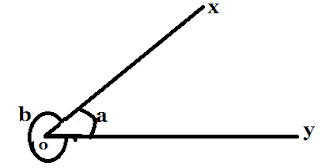In the above figure angle XON= b˚ is the reflex angle.

Complete angle: An angle whose value is 360˚ is known as complete angle.
Complimentary angle: If the sum of Two angles is 90˚ then they are known as complimentary angle.
Ex: If angle A =50˚ is compliment to angle B then the value of B=(90˚- 50˚)=40˚
Supplementary angle: If the sum of the angles is 180˚ then they are known as supplementary angle.
Adjacent Angles: Angles having one side as common is known as adjacent angles.Here angles a,b are having a common side and thus these are known as adjacent angles.
Linear Pair angles: Two angles form a linear if they are having common vertex and sum of the angles is 180˚.
Note: All adjacent angles are not linear pair angles.
Ex: In the given figure angle XOZ and YOZ form a linear pair if a-b=80˚ find the value of a and b.Given a-b=80˚
a+b=180˚ as it is clearly stated that a and b are linear pair.
BY solving both the equations we get ,
2a=260˚,
a=130˚ and the value of b=50˚

#### VERTICALLY OPPOSITE ANGLES:

When two lines intersect each other four angles are formed . The angles lie on the opposite side of the intersecting point are called as vertically opposite angles.Here angles p and q are opposite to each other similarly s and r are opposite to each other.
Vertically opposite angles are always equal i.e
p=q
r=s.

Angle Bisector: A line which cuts angle into two equal angles is called an angle bisector. An angle bisector can be of two types
1.Internal angle bisector
2. External angle bisector.

#### Internal angle Bisector:From the above figure it is clear that what is meant by a angle bisector. or is an angle bisector which divided the angle qos into two equal angles.

## Triangle:

A plane figure bounded by three straight lines is called a triangle.The above figure shows how a triangle looks like.

#### Types Of Triangles: Based on Angles

Acute angled Triangle: A triangle which each angle is less than 90˚ is called as acute angled triangle.
Right angled Traingle: A triangle in which one of the three angles Is 90˚ is known as right angled triangle.
Obtuse angled Triangle: A triangle in which one of the angle lies between 90˚ and 180˚ is known as obtuse angled triangle.

#### Types of Triangle: Based on Sides

Equilateral Triangle: A triangle in which all the three sides are equal is known as equilateral triangle.
Scalene Triangle: A triangle in which all the three sides are of different length is known as scalene triangle.
Isosceles Triangle: A triangle in which two out of three sides are equal is known as Isosceles triangle.

#### Congruence of Triangle:

In Geometry, two figures are congruent or similar if they have same size and shape.
Two line segments are said to be congruent if they have same length
Two angles are congruent if they have same measure
Two circles are congruent if they have same diameter
In the same way, two triangles can be congruent in the following cases
SSS: In this case, if three sides of one triangle are equal to the three sides of another triangle then they are said to be congruent.
SAS: In this case, if two sides and an angle made by one triangle is equal to the two sides and angle made by another triangle then they are said to be congruent.
ASA: Two angles and a side of a triangle is equal to two angles and a side of another triangle then the triangles are said to be congruent.

If the corresponding angles of two triangles are equal and differ in the length of the sides. Then
Let us discuss more properties of triangle, orthocenter, incenter, polygons in the next article….ss